• 2021-05-31 12:35:22

# A算法与D算法

#### 1. A* (Informed Search)

1.1

Evaluation Function: f(n)=g(n)+h(n)
operating cost function: g(n)
Heuristic fucntion: h(n)
（相较于Dijkstra 算法 增加了启发式函数 来确定最优路径）
可用启发式函数：曼哈顿距离 欧式距离 对角距离

流程图如下图所示：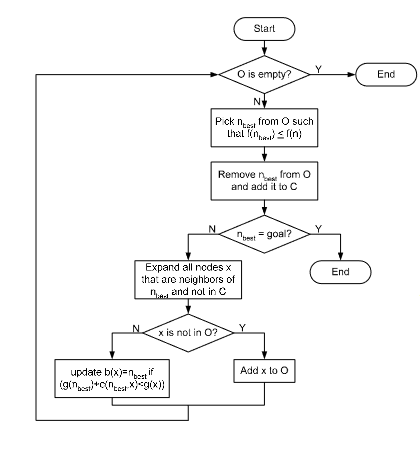Open list： 存储扩展的节点
close list: 存储已经搜索过的节点

1.2 A*算法运行中需要注意的问题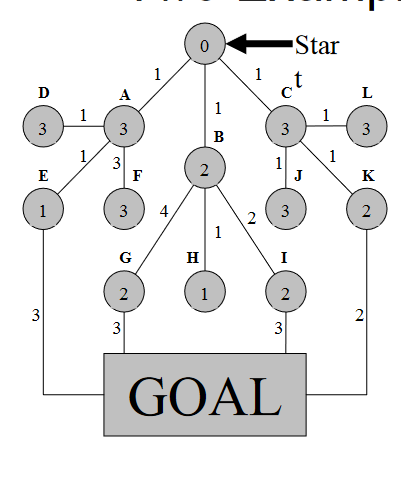• 从root开始， 圆圈内表示该节点启发式函数数值，连线上表示代价函数数值
• 每一个节点的启发式函数是独立的，不与之前节点启发式函数大小相关
• 途中节点的代价函数数值总是由root 出发的代价函数总和
• 每一步搜索时将open list中f(n)最小的节点添加到close list 中，要注意close list中要带上f(n)值进行存储，以便于最终到达goal时判断最优路径
• close list中出现goal节点即为停止搜索flag

1.3 A* replanner (用于未知地图情况)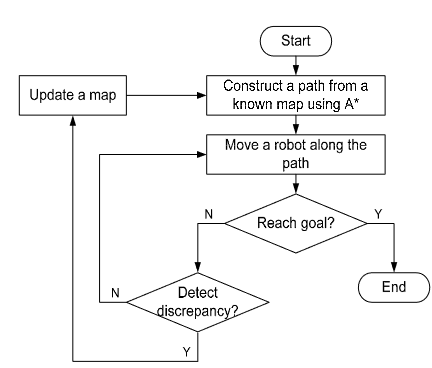• 流程右半部分为正常A* 流程， 从ROOT出发在已知地图中进行搜索，判断是否到达GOAL
• 如果没有到达GOAL，则判断是否与一直地图存在差异
• 若与已知地图存在差异，则更新地图，并使用更新后地图继续搜索
• 若与已知地图没有差异，说明没有动态障碍物产生，继续按照原地图搜索
• 知道到达GOAL停止

#### 2. D*算法 （Stentz 1994）

2.1 介绍

• 又称动态A搜索 (Dynamic A Search)
• 过程中节点间代价函数会发生改变 (replanning online)
• 等价于A* replanner
• 使用Dijkstra 进行初始规划，并通过接受实时数据来加快规划速度，针对部分地图区域来实现动态避障
• 比A* replanner 更高效 (大范围复杂环境扩展中)
• 用于在第一次逆向搜索获得最优路径并记录节点间关系后，处理动态问题
• h：到GOAL代价 k:该点最小的h
• 使用k原因：k用于open list排序，说明此节点之前为最优节点，可在此节点周围进行搜索，加快重新规划的速度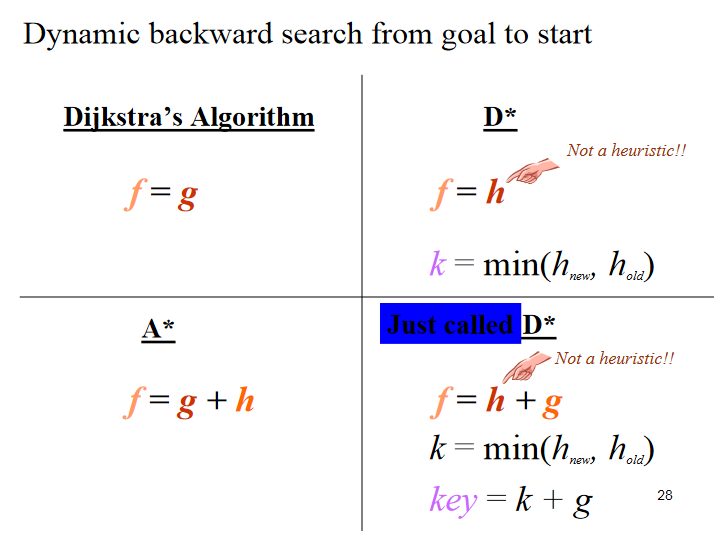2.2 步骤

• 初始阶段，所有节点均为NEW（代表第一次遍历到），h和k值均为到GOAL的距离
• 从GOAL出发，将GOAL添加到open list中，且它的h=0 k=0 (k值为队列中优先度)
• 将GOAL pop出open list，扩展到GOAL的相邻节点，因为此时节点均为NEW，所以k=h=到GOAL的距离，将相邻节点推入open list中
• 不停寻找相邻节点，迭代，推入推出open list
• !!!若此时遇到节点处为障碍物，此节点h值很高，因为为NEW，所以k=h=high value
• 当root被扩展后，则搜索结束
• 根据h的梯度确定最优路径 （方向指向临间节点中k值最小的节点）
• ！！当计划路径上某点被阻碍，因为是反向指针，可以定位到被堵节点的上一节点（D*速度快的原因）
• 障碍物节点h升高，RISE态，需搜索使得该点的h降低，回复lower state
• 将该障碍点和临间节点加入open list中，进行搜索，如果无法使该点转为lower态，说明临间通过节点无法成功绕开障碍物，则将该影响扩散
• 该点的子节点受到影响，h变大，并进行判断
• 若 k_old<h(x): 该节点x处于raise状态，如果在x周围找到一个临间节点，使得h.y+c（x,y）更小，那就修改x的父节点，重置其h的值（父节点h+节点间距离）。
• 若k_old=h(x): 该节点x处于lower的状态，并没有受到障碍影响，或者还在第一遍遍历的阶段。
更多相关内容
• A*算法，动态路径规划算法的一种，程序直接放到matlab即可运行。
• a*算法流程图(只是流程图)A*算法是一种在静态路网中求解最短路径最有效的直接搜索方法，也是解决许多其他搜索问题的有效算法算法中的距离估算值与实际值越接近，扩展的节点数越少， 搜索速度越快。
• A*算法进行二维路径规划，即AStar算法， matlab程序，可以直接运行
• 背景：项目需要接触此算法，以下是一些自学成果，如有不足之处，欢迎指出，必虚心接受。做了一份PPT来汇报，此处直接使用自己PPT的截图。部分图片来源网络，如有侵权立马删除，以下博文仅作为学习...A*算法的定义伪...

背景：项目需要接触此算法，以下是一些自学成果，如有不足之处，欢迎指出，必虚心接受。做了一份PPT来汇报，此处直接使用自己PPT的截图。部分图片来源网络，如有侵权立马删除，以下博文仅作为学习笔记。后期又新增了完整PPT。A*算法完整PTT_dujuancao11的博客-CSDN博客

目录

A*寻路算法

A*算法解决什么问题

A*算法的基本原理

A*算法的详细原理

A*算法的详细原理之定义

​A*算法的详细原理之初始设定

​A*算法的详细原理之寻路原理

A*算法的详细原理之结束条件

A*算法的寻路详细步骤

A*算法的举例说明

A*算法的伪代码

A*算法的定义伪代码 (C++)

A*算法的寻路伪代码(C++)

Python+PyQt代码实现

代码内容（可运行）

运行结果

可执行文件

拓展

Dijkstra算法和A*算法的比较

# A*寻路算法

## A*算法解决什么问题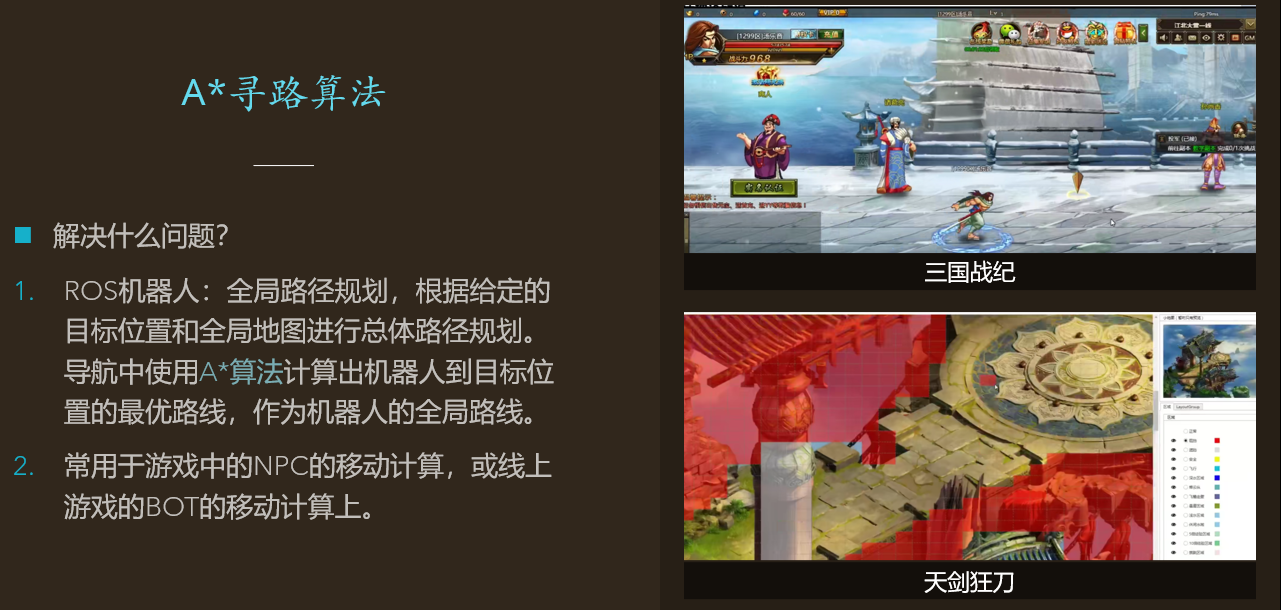## A*算法的基本原理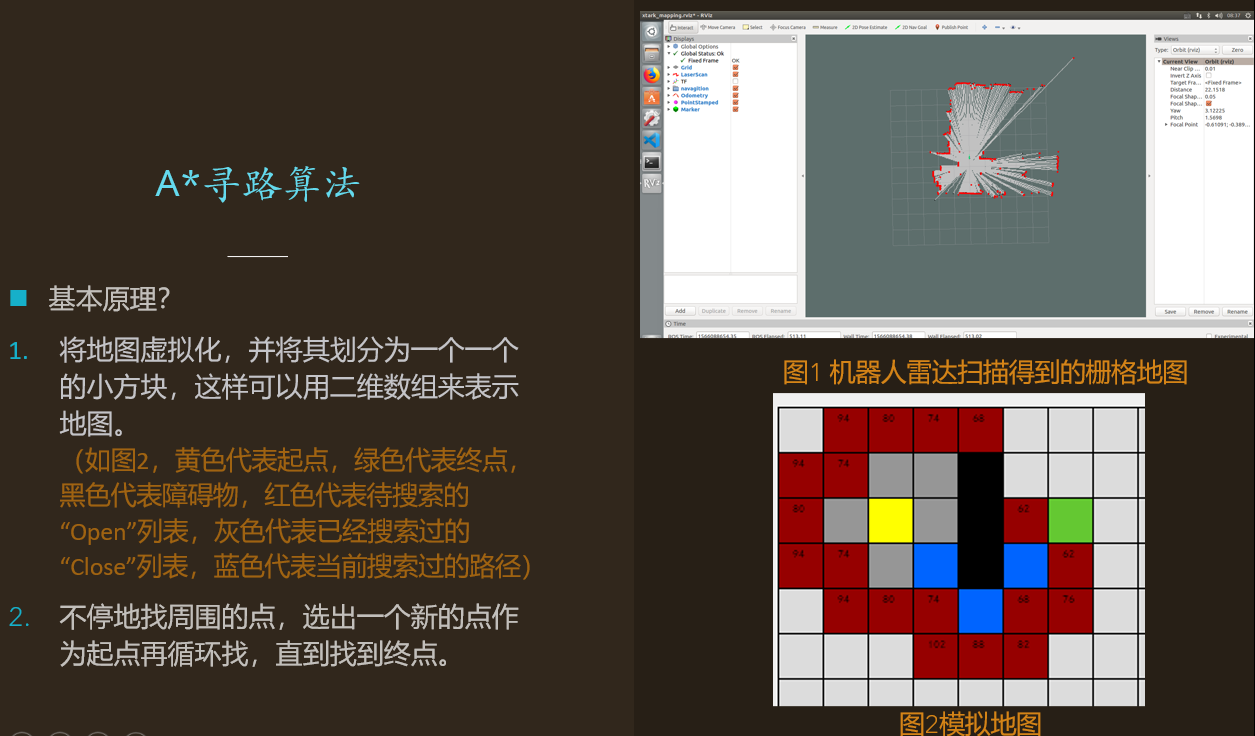## A*算法的详细原理

###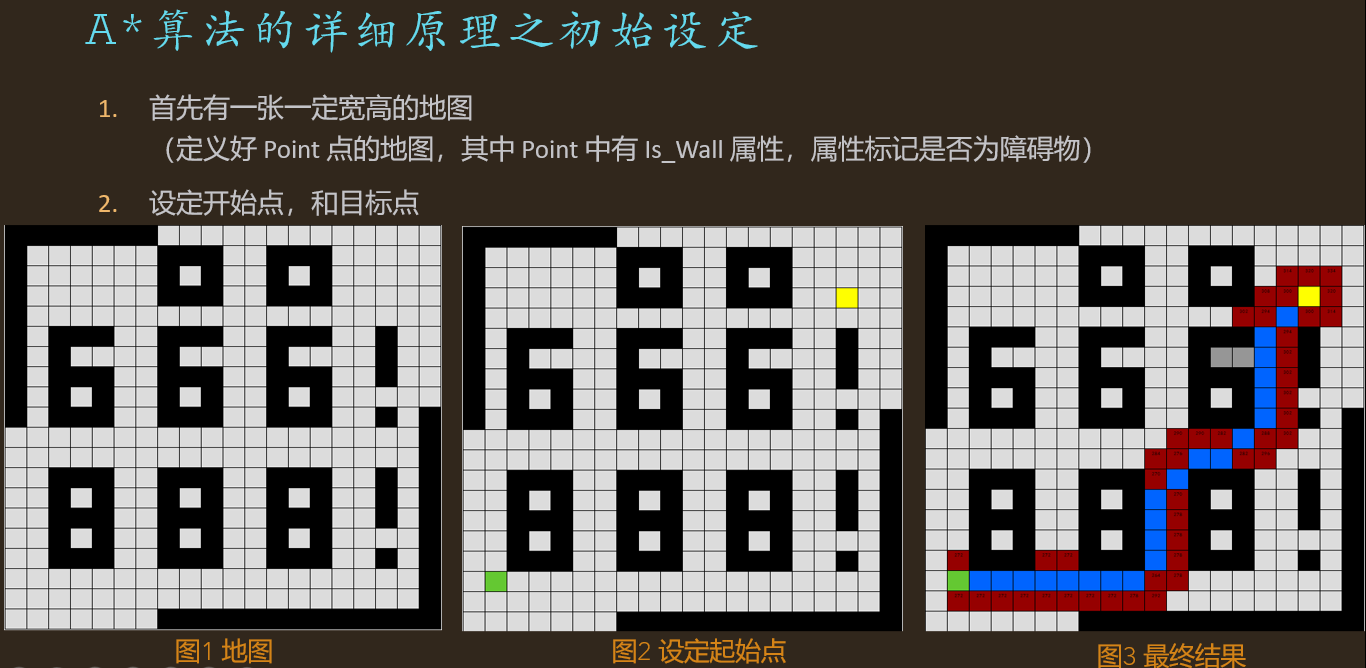A*算法的详细原理之寻路原理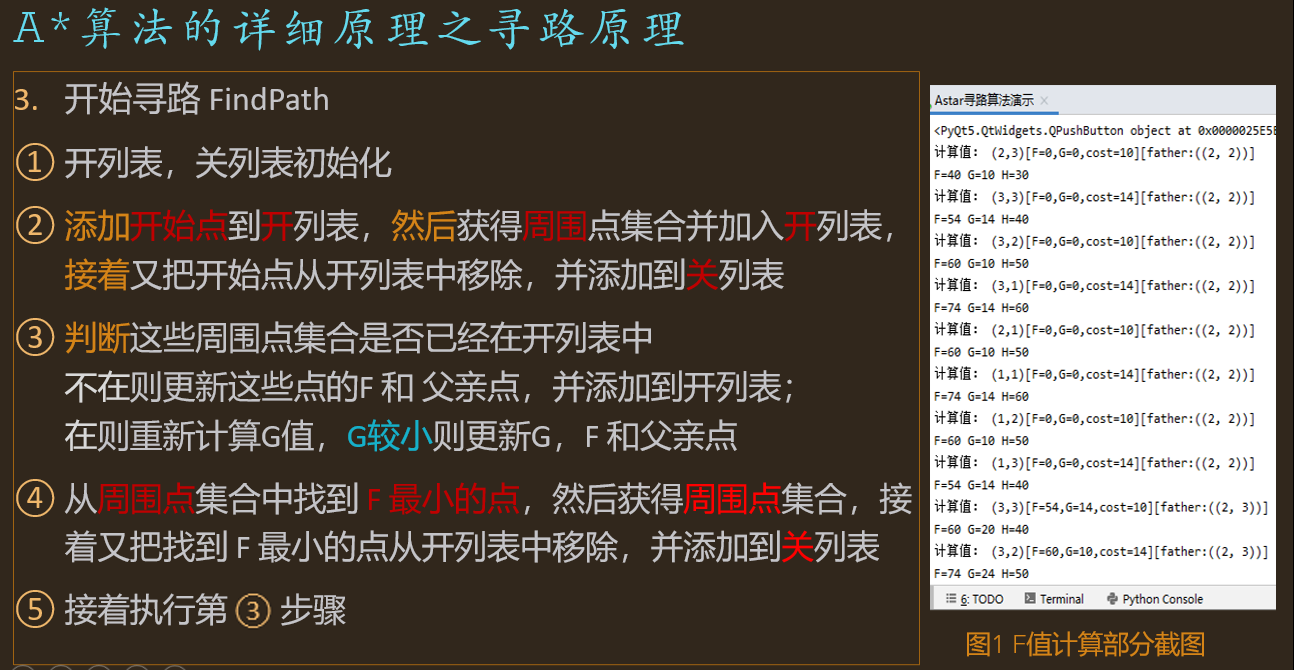### A*算法的详细原理之结束条件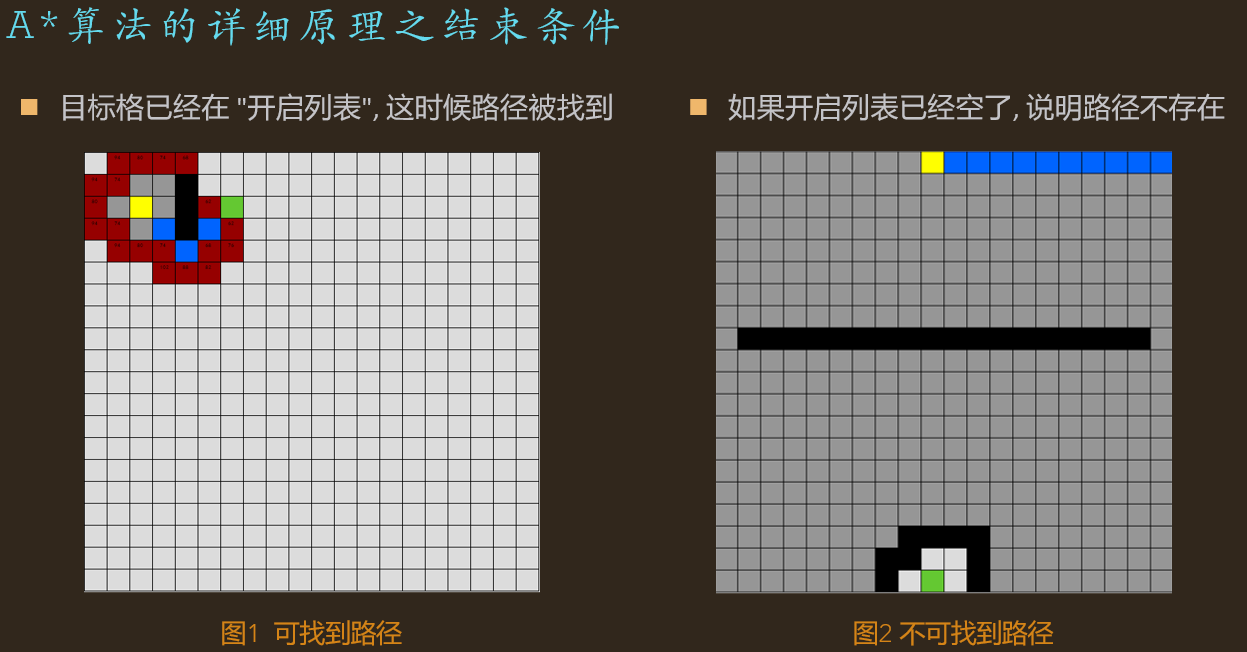## A*算法的寻路详细步骤

S（start）起点              E（End）终点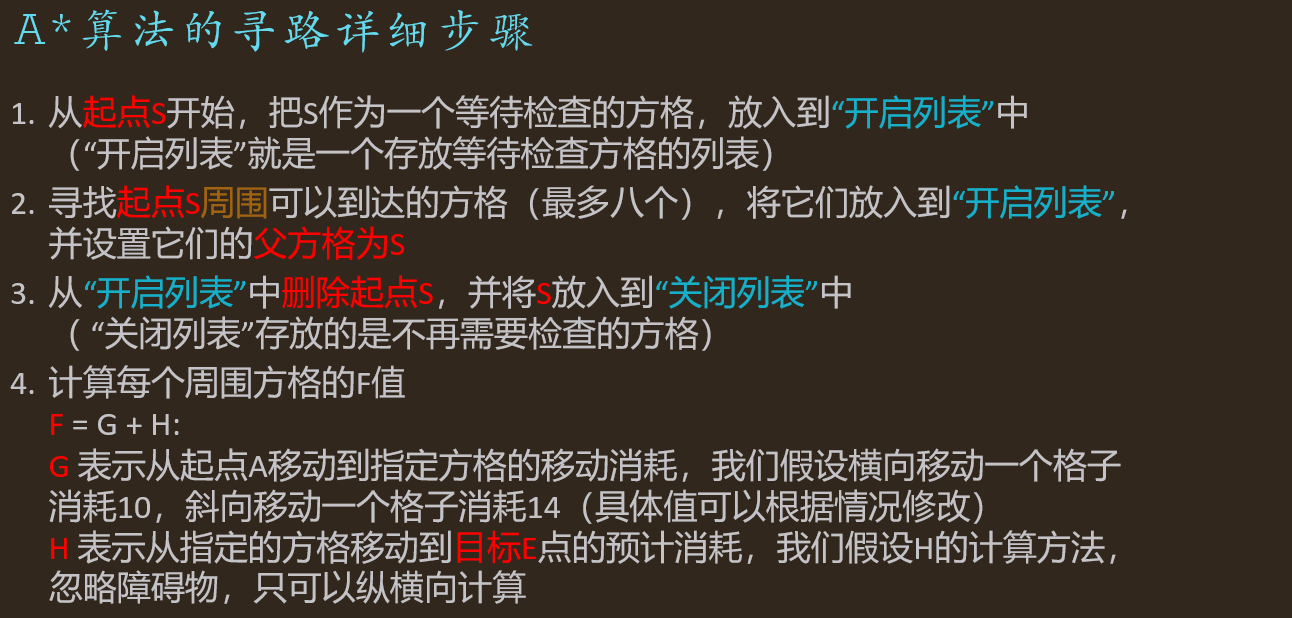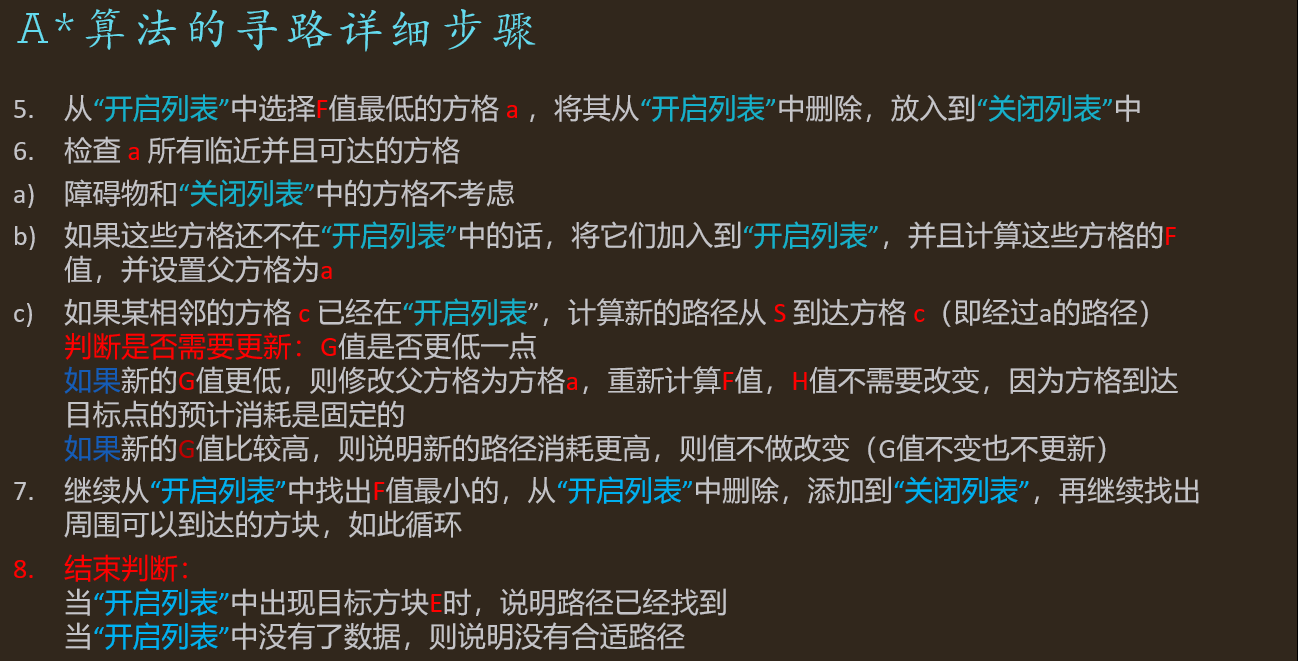## A*算法的举例说明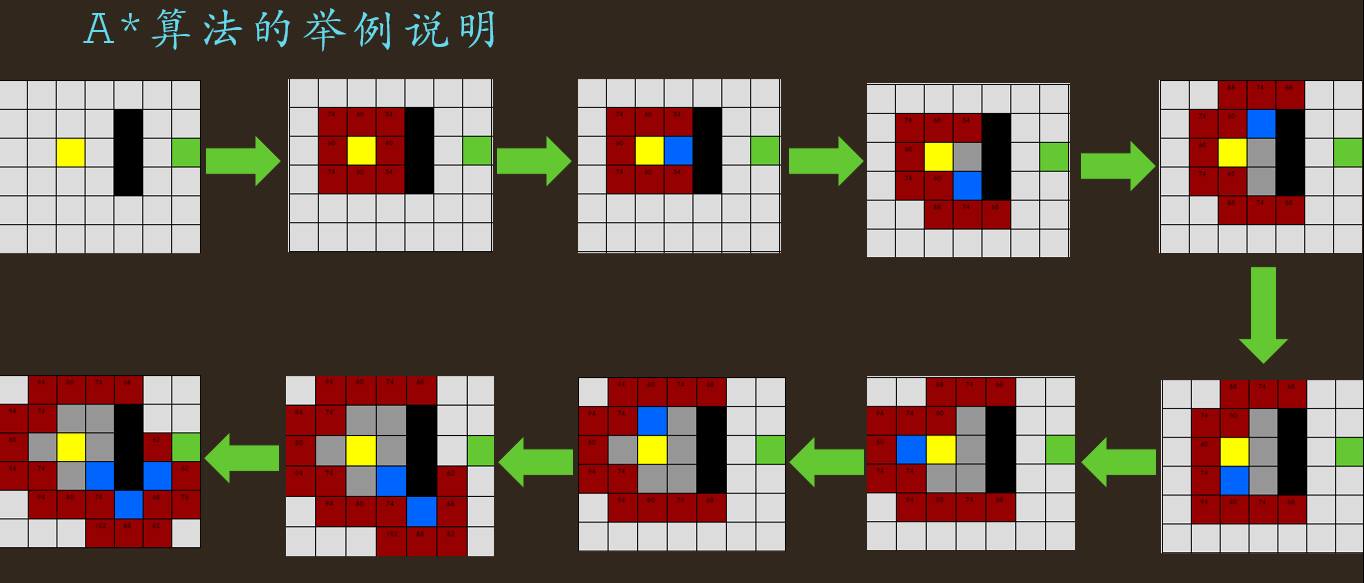如果还不懂的话，可以参考我手写的计算步骤，再不懂可以私信我。（手稿字有点丑）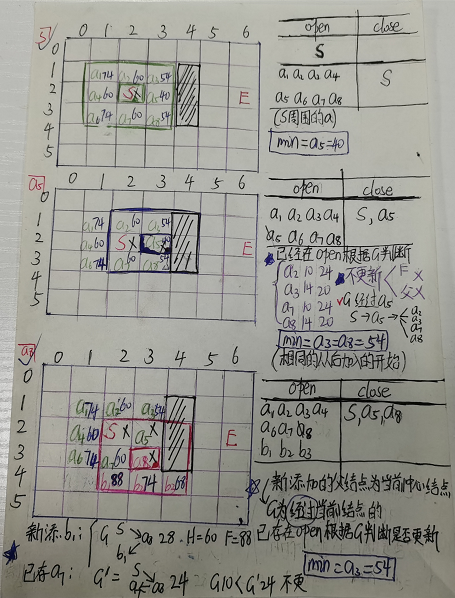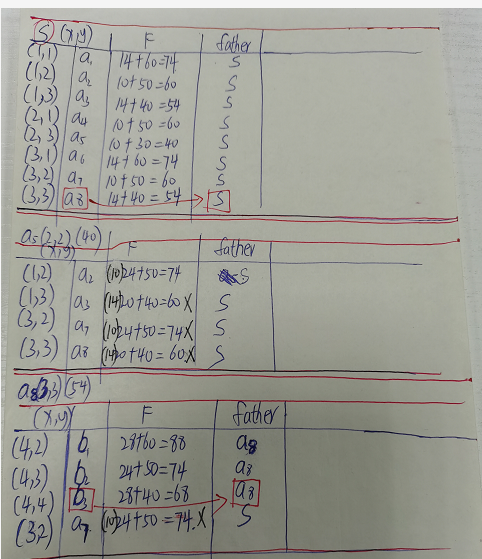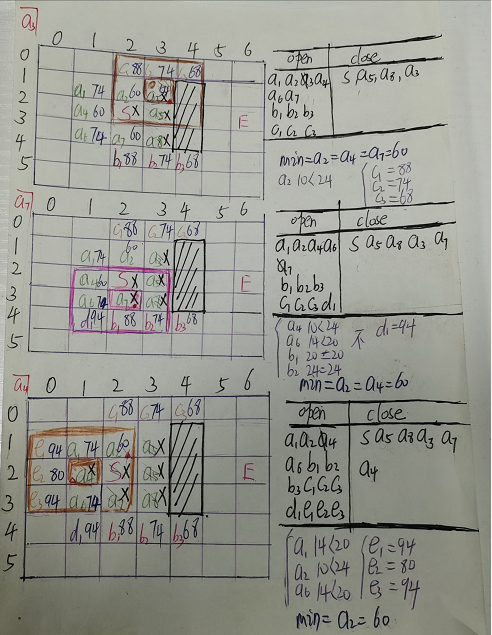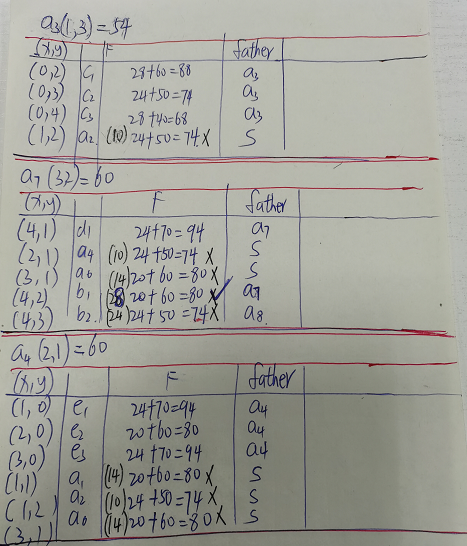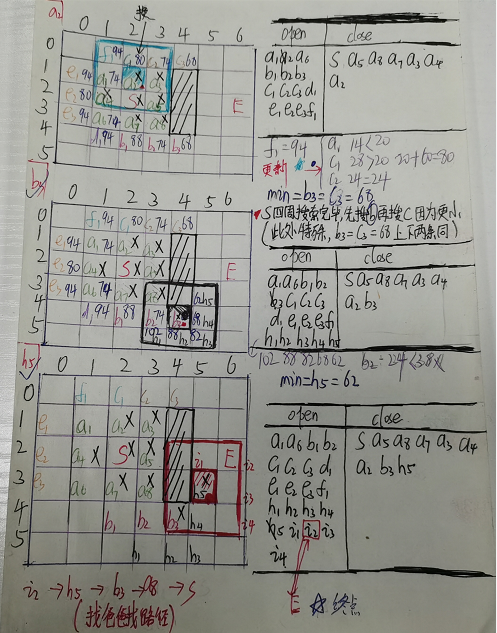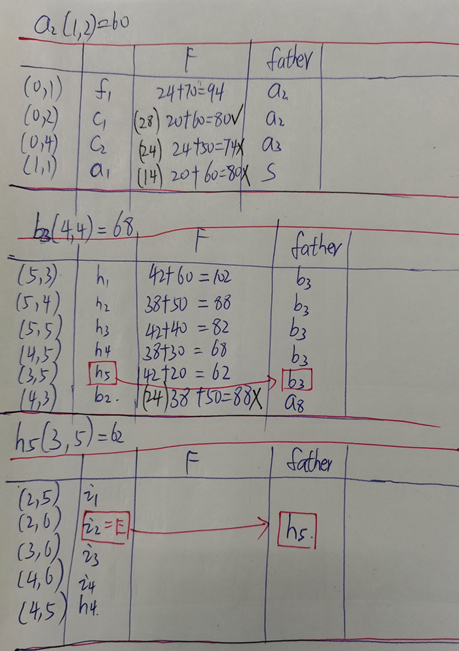## A*算法的伪代码

### A*算法的定义伪代码 (C++)

int open_list;//一个记录下所有被考虑来寻找最短路径的格子
int close_list; //一个记录下不会再被考虑的格子
typedef struct point{
bool Is_Wall;
struct point* father;//父节点
int G;// 表示从起点 A 移动到网格上指定方格的移动耗费 (上下左右，还可沿斜方向移动)
int old_G;//旧G 第一次：从起点 A 直接移动到 A 四周方格的移动耗费 ；上次更新得到的G
int new_G; //新G  从起点 A 经过当前搜索中心点到其四周指定点的移动耗费
int H;//表示从指定的方格移动到终点 B 的预计耗费 (H 有很多计算方法, 这里我们设定只可以上下左右移动)
int F=G+H;//表示该点的总耗费
}Point;
point* start_point;
point* end_point;
point* min_point;
point* now_point; 

### A*算法的寻路伪代码(C++)

//FindPath
do{
//确定中心搜索点，上一个中心点关闭，新的中心点开启
查找：Find the minimumm "point" of "F" from the "open_list" center;
"now_point" = "min_point";//minimumm point
"now_point"添加到"close_list";

//新中心点的周围点开启，新中心点关闭
循环遍历："now_point"相邻的周围8格"s_now_point"中的每一个;
//这一块它指的就是now_point周围8点当前搜索点 s_now_point，为了简单直接用它表示
if (它不可通过||它已经在"close_list"中){
什么也不做;
} else if (它不在开启列表中){
把它添加进"open_list";
把"now_point"作为这它的"father",计算它的"F","G","H";
}else if (它已经在开启列表中){//通过G来判断是否需要更新
if (new_G < old_G){
更新它的"father"为当前中心搜索点"now_point";
更新它的"G"与"F" ;
} else{
不更新，保持原来的"father", "G"与"F" ;
}
}
} while(目标格"end_point"已经在"open_list"||"open_list"==NULL)
//存在路径：目标格"end_point"已经在"open_list"
//不存在路径： "open_list"==NULL，搜索了所有可能的点


## Python+PyQt代码实现

### 代码内容（可运行）

import time,sys
from PyQt5.QtWidgets import QDialogButtonBox,QDialog,QMainWindow,QGridLayout,QTextEdit,QLineEdit,QWidget, QMessageBox, QApplication,QLabel,QPushButton,QHBoxLayout,QVBoxLayout
from PyQt5.QtCore import Qt,QTimer,QObject,pyqtSignal,QBasicTimer
from PyQt5.QtGui import QPainter, QColor, QFont,QPen
import json
class config:
WIDTH=20#地图列数
HEIGHT=20#地图行数
blockLength=30#绘制画面时每一个节点方块的边长
class point:#点类（每一个唯一坐标只有对应的一个实例）
_list=[]#储存所有的point类实例
_tag=True#标记最新创建的实例是否为_list中的已有的实例，True表示不是已有实例
def __new__(cls,x,y):#重写new方法实现对于同样的坐标只有唯一的一个实例
for i in point._list:
if i.x==x and i.y==y:
point._tag=False
return i
nt=super(point,cls).__new__(cls)
point._list.append(nt)
return nt
def __init__(self,x,y):
if point._tag:
self.x=x
self.y=y
self.father=None
self.F=0#当前点的评分  F=G+H
self.G=0#起点到当前节点所花费的消耗
self.cost=0#父节点到此节点的消耗
else:
point._tag=True
@classmethod
def clear(cls):#clear方法，每次搜索结束后，将所有点数据清除，以便进行下一次搜索的时候点数据不会冲突。
point._list=[]
def __eq__(self,T):#重写==运算以便实现point类的in运算
if type(self)==type(T):
return (self.x,self.y)==(T.x,T.y)
else:
return False
def __str__(self):
return'(%d,%d)[F=%d,G=%d,cost=%d][father:(%s)]'%(self.x,self.y,self.F,self.G,self.cost,str((self.father.x,self.father.y)) if self.father!=None else 'null')
class A_Search:#核心部分，寻路类
def __init__(self,arg_start,arg_end,arg_map):
self.start=arg_start#储存此次搜索的开始点
self.end=arg_end#储存此次搜索的目的点
self.Map=arg_map#一个二维数组，为此次搜索的地图引用
self.open=[]#开放列表：储存即将被搜索的节点
self.close=[]#关闭列表：储存已经搜索过的节点
self.result=[]#当计算完成后，将最终得到的路径写入到此属性中
self.count=0#记录此次搜索所搜索过的节点数
self.useTime=0#记录此次搜索花费的时间--在此演示中无意义，因为process方法变成了一个逐步处理的生成器，统计时间无意义。
#开始进行初始数据处理
self.open.append(arg_start)
def cal_F(self,loc):
print('计算值：',loc)
G=loc.father.G+loc.cost
H=self.getEstimate(loc)
F=G+H
print("F=%d G=%d H=%d"%(F,G,H))
return {'G':G,'H':H,'F':F}
def F_Min(self):#搜索open列表中F值最小的点并将其返回，同时判断open列表是否为空，为空则代表搜索失败
if len(self.open)<=0:
return None
t=self.open
for i in self.open:
if i.F<t.F:
t=i
return t
def getAroundPoint(self,loc):#获取指定点周围所有可通行的点，并将其对应的移动消耗进行赋值。
l=[(loc.x,loc.y+1,10),(loc.x+1,loc.y+1,14),(loc.x+1,loc.y,10),(loc.x+1,loc.y-1,14),(loc.x,loc.y-1,10),(loc.x-1,loc.y-1,14),(loc.x-1,loc.y,10),(loc.x-1,loc.y+1,14)]
for i in l[::-1]:
if i<0 or i>=config.HEIGHT or i<0 or i>=config.WIDTH:
l.remove(i)
nl=[]
for i in l:
if self.Map[i][i]==0:
nt=point(i,i)
nt.cost=i
nl.append(nt)
return nl

for i in l:
if i not in self.open:
if i not in self.close:
i.father=father
self.open.append(i)
r=self.cal_F(i)
i.G=r['G']
i.F=r['F']
else:
tf=i.father
i.father=father
r=self.cal_F(i)
if i.F>r['F']:
i.G=r['G']
i.F=r['F']
# i.father=father
else:
i.father=tf
def getEstimate(self,loc):#H :从点loc移动到终点的预估花费
return (abs(loc.x-self.end.x)+abs(loc.y-self.end.y))*10
def DisplayPath(self):#在此演示中无意义
print('搜索花费的时间:%.2fs.迭代次数%d,路径长度:%d'%(self.useTime,self.count,len(self.result)))
if self.result!=None:
for i in self.result:
self.Map[i.x][i.y]=8
for i in self.Map:
for j in i:
if j==0:
print('%s'%'□',end='')
elif j==1:
print('%s'%'▽',end='')
elif j==8:
print('%s'%'★',end='')
print('')
else:
print('搜索失败，无可通行路径')
def process(self):#使用yield将process方法变成一个生成器，可以逐步的对搜索过程进行处理并返回关键数据
while True:
self.count+=1
tar=self.F_Min()#先获取open列表中F值最低的点tar
if tar==None:
self.result=None
self.count=-1
break
else:
aroundP=self.getAroundPoint(tar)#获取tar周围的可用点列表aroundP
self.open.remove(tar)#将tar从open列表中移除
self.close.append(tar)#已经迭代过的节点tar放入close列表中
if self.end in self.open:#判断终点是否已经处于open列表中
e=self.end
self.result.append(e)
while True:
e=e.father
if e==None:
break
self.result.append(e)
yield (tar,self.open,self.close)
break

# self.repaint()
# print('返回')
yield (tar,self.open,self.close)
time.sleep(5)#暂停
self.useTime=time2-time1
class GameBoard(QMainWindow):#可视化类，pyqt5进行编写。
def __init__(self):
print('初始化地图...')
self.Map=[]
for i in range(config.HEIGHT):
col=[]
for j in range(config.WIDTH):
col.append(0)
self.Map.append(col)
self.startPoint=None
self.endPoint=None
self.search=None
self.centerTimer=None
self.yi=None
self.special=None
self.displayFlush=False
super().__init__()
print('初始化UI...')
self.initUI()
def initUI(self):
#开始初始化UI部分
#创建UI控件
self.label_tips=QLabel("<p style='color:green'>使用说明：</p>右键单击格子选定起始点,左键格子选定格子为墙壁或删除墙壁。\n<p style='color:green'>颜色说明：</p>\n黄色代表起点，绿色代表终点，黑色代表墙壁，红色代表待搜索的open列表，灰色代表已搜索过的close列表，蓝色代表当前搜索到的路径",self)
self.label_display=QLabel("",self)
self.button_start=QPushButton("开始搜索",self)
self.button_clearSE=QPushButton("重选起始点",self)
self.button_clearWall=QPushButton("清空地图墙壁",self)
self.button_saveMap=QPushButton("保存地图",self)

#设置控件属性
self.label_tips.setWordWrap(True)
self.label_display.setWordWrap(True)
#设置控件样式
self.label_display.setStyleSheet("border:1px solid black")
self.label_display.setAlignment(Qt.AlignLeft)
self.label_display.setAlignment(Qt.AlignTop)
#设置控件的尺寸和位置
self.label_tips.resize(200,150)
self.button_saveMap.resize(80,30)
self.label_display.resize(200,300)

self.label_tips.move(100+(config.WIDTH-1)*config.blockLength,0)
self.label_display.move(100+(config.WIDTH-1)*config.blockLength,400)
self.button_start.move(100+(config.WIDTH-1)*config.blockLength,200)
self.button_clearSE.move(100+(config.WIDTH-1)*config.blockLength,250)
self.button_clearWall.move(100+(config.WIDTH-1)*config.blockLength,300)
self.button_saveMap.move(100+(config.WIDTH-1)*config.blockLength,350)
#给控件绑定事件
self.button_start.clicked.connect(self.button_StartEvent)
self.button_clearSE.clicked.connect(self.button_Clear)
self.button_clearWall.clicked.connect(self.button_Clear)
self.button_saveMap.clicked.connect(self.button_SaveMap)
#UI初始化完成
self.setGeometry(0, 0, 150+(config.WIDTH*config.blockLength-config.blockLength)+200, 150+(config.HEIGHT*config.blockLength-config.blockLength))
self.setMinimumSize(150+(config.WIDTH*config.blockLength-config.blockLength)+200, 150+(config.HEIGHT*config.blockLength-config.blockLength))
self.setMaximumSize(150+(config.WIDTH*config.blockLength-config.blockLength)+200, 150+(config.HEIGHT*config.blockLength-config.blockLength))
self.setWindowTitle('A*搜索')
self.show()
if self.displayFlush:
self.label_display.setText(text+'\n')
self.displayFlush=False
else:
self.label_display.setText(self.label_display.text()+text+'\n')
def mousePressEvent(self,event):
x,y=event.x()-50,event.y()-50
x=x//config.blockLength
y=y//config.blockLength
if x>=0 and x<config.WIDTH and y>=0 and y<config.HEIGHT:
if event.button()==Qt.LeftButton:
if (x,y)!=self.startPoint and (x,y)!=self.endPoint:
self.Map[y][x]=(1 if self.Map[y][x]==0 else 0)
if event.button()==Qt.RightButton:
if self.Map[y][x]==0:
if self.startPoint==None:
self.startPoint=(x,y)
elif self.endPoint==None and self.startPoint!=(x,y):
self.endPoint=(x,y)
self.repaint()
def button_StartEvent(self):
sender=self.sender()
print(sender)
if self.startPoint!=None and self.endPoint!=None:
if self.centerTimer==None:
self.centerTimer=QBasicTimer()
self.button_start.setEnabled(False)
self.button_clearSE.setEnabled(False)
self.button_clearWall.setEnabled(False)
self.centerTimer.start(200,self)
self.search=A_Search(point(self.startPoint,self.startPoint),point(self.endPoint,self.endPoint),self.Map)
self.yi=self.search.process()
def button_SaveMap(self):
with open('map.txt','w') as f:
f.write(json.dumps(self.Map))
# else:
try:
with open('map.txt','r') as f:
config.HEIGHT=len(self.Map)
config.WIDTH=len(self.Map)
self.repaint()
except Exception as e:
print('失败',e,type(e))
if type(e)==FileNotFoundError:
elif type(e)==json.decoder.JSONDecodeError:
def button_Clear(self):
sender=self.sender()
print(self.button_clearSE,type(self.button_clearSE))
if sender==self.button_clearSE:
self.startPoint=None
self.endPoint=None
self.repaint()
elif sender==self.button_clearWall:
for i in range(len(self.Map)):
for j in range(len(self.Map[i])):
self.Map[i][j]=0
self.repaint()
def paintEvent(self, event):
qp = QPainter()
qp.begin(self)
self.drawBoard(event,qp)
qp.end()
def drawBoard(self, event, qp):
self.drawMap(qp)
def drawMap(self,qp):#画面绘制方法，每次地图有所改动都将重绘
time1=time.time()
if self.search!=None:
if self.special!=None:
e=self.special
path=[e]
while True:
e=e.father
if e!=None:
path.append(e)
else:
break
else:
path=None
pen=QPen(QColor(0,0,0),1,Qt.SolidLine)
qp.setPen(pen)
for i in range(len(self.Map)):
for j in range(len(self.Map[i])):
wordTag=False
if i==self.search.start.x and j==self.search.start.y:
qp.setBrush(QColor(255,255,0))
elif i==self.search.end.x and j==self.search.end.y:
qp.setBrush(QColor(100,200,50))
else:
if self.Map[i][j]==0:
tagx=True
if path:
for k in path:
if k.x==i and k.y==j:
tagx=False
qp.setBrush(QColor(0,100,255))
if tagx:
if self.special!=None:
if i==self.special.x and j==self.special.y:
qp.setBrush(QColor(0,255,0))
else:
tag=True
for k in self.special:
if k.x==i and k.y==j:
tag=False
wordTag=True
word=str(k.F)

qp.setBrush(QColor(150,0,0))
break
else:
qp.setBrush(QColor(220,220,220))
if tag:
for k in self.special:
if k.x==i and k.y==j:
qp.setBrush(QColor(150,150,150))
break
else:
qp.setBrush(QColor(220,220,220))
else:
qp.setBrush(QColor(220,220,220))
elif self.Map[i][j]==1:
qp.setBrush(QColor(0,0,0))
else:
qp.setBrush(QColor(255,0,0))
qp.drawRect(50+j*config.blockLength,50+i*config.blockLength,config.blockLength,config.blockLength)
if wordTag:
qp.setFont(QFont('楷体',5,QFont.Light))
qp.drawText(50+10+j*config.blockLength,50+10+i*config.blockLength,word)
wordTag=False
#time.sleep(20)
else:
for i in range(len(self.Map)):
for j in range(len(self.Map[i])):
if (j,i)==self.startPoint:
qp.setBrush(QColor(255,255,0))
elif (j,i)==self.endPoint:
qp.setBrush(QColor(100,200,50))
else:
if self.Map[i][j]==0:
qp.setBrush(QColor(220,220,220))
elif self.Map[i][j]==1:
qp.setBrush(QColor(0,0,0))
else:
qp.setBrush(QColor(255,0,0))

qp.drawRect(50+j*config.blockLength,50+i*config.blockLength,config.blockLength,config.blockLength)
time2=time.time()
#time.sleep(20)
# print('绘制时间：',time2-time1)
def timerEvent(self,e):
try:
data=next(self.yi)
except Exception as e:
print('搜索结束！')
if self.search.result==None:
print('搜索结束！')
else:
self.centerTimer.stop()
self.search=None
self.yi=None
self.special=None
point.clear()
self.button_start.setEnabled(True)
self.button_clearSE.setEnabled(True)
self.button_clearWall.setEnabled(True)
self.displayFlush=True
else:
self.special=data
self.repaint()
if __name__ == '__main__':
app = QApplication(sys.argv)
ex = GameBoard()
sys.exit(app.exec_())



注意：代码运行可以设置动态遍历的时候暂停时间（大概在145行的time.sleep(5)语句)

### 运行结果

输出每次计算的每个点的F和父结点，直接看图吧！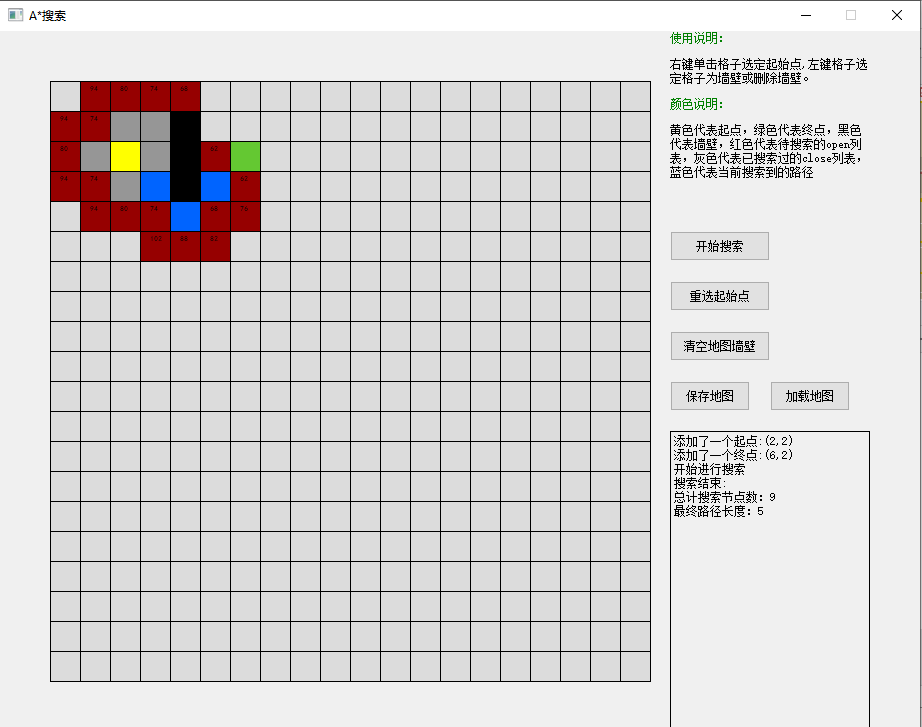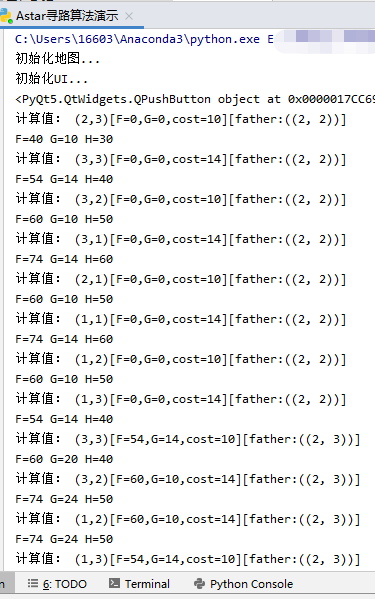详细列表

初始化地图...
初始化UI...
<PyQt5.QtWidgets.QPushButton object at 0x0000017CC699AC18>
计算值： (2,3)[F=0,G=0,cost=10][father:((2, 2))]
F=40 G=10 H=30
计算值： (3,3)[F=0,G=0,cost=14][father:((2, 2))]
F=54 G=14 H=40
计算值： (3,2)[F=0,G=0,cost=10][father:((2, 2))]
F=60 G=10 H=50
计算值： (3,1)[F=0,G=0,cost=14][father:((2, 2))]
F=74 G=14 H=60
计算值： (2,1)[F=0,G=0,cost=10][father:((2, 2))]
F=60 G=10 H=50
计算值： (1,1)[F=0,G=0,cost=14][father:((2, 2))]
F=74 G=14 H=60
计算值： (1,2)[F=0,G=0,cost=10][father:((2, 2))]
F=60 G=10 H=50
计算值： (1,3)[F=0,G=0,cost=14][father:((2, 2))]
F=54 G=14 H=40
计算值： (3,3)[F=54,G=14,cost=10][father:((2, 3))]
F=60 G=20 H=40
计算值： (3,2)[F=60,G=10,cost=14][father:((2, 3))]
F=74 G=24 H=50
计算值： (1,2)[F=60,G=10,cost=14][father:((2, 3))]
F=74 G=24 H=50
计算值： (1,3)[F=54,G=14,cost=10][father:((2, 3))]
F=60 G=20 H=40
计算值： (4,4)[F=0,G=0,cost=14][father:((3, 3))]
F=68 G=28 H=40
计算值： (4,3)[F=0,G=0,cost=10][father:((3, 3))]
F=74 G=24 H=50
计算值： (4,2)[F=0,G=0,cost=14][father:((3, 3))]
F=88 G=28 H=60
计算值： (3,2)[F=60,G=10,cost=10][father:((3, 3))]
F=74 G=24 H=50
计算值： (1,2)[F=60,G=10,cost=10][father:((1, 3))]
F=74 G=24 H=50
计算值： (0,2)[F=0,G=0,cost=14][father:((1, 3))]
F=88 G=28 H=60
计算值： (0,3)[F=0,G=0,cost=10][father:((1, 3))]
F=74 G=24 H=50
计算值： (0,4)[F=0,G=0,cost=14][father:((1, 3))]
F=68 G=28 H=40
计算值： (4,3)[F=74,G=24,cost=14][father:((3, 2))]
F=74 G=24 H=50
计算值： (4,2)[F=88,G=28,cost=10][father:((3, 2))]
F=80 G=20 H=60
计算值： (4,1)[F=0,G=0,cost=14][father:((3, 2))]
F=94 G=24 H=70
计算值： (3,1)[F=74,G=14,cost=10][father:((3, 2))]
F=80 G=20 H=60
计算值： (2,1)[F=60,G=10,cost=14][father:((3, 2))]
F=74 G=24 H=50
计算值： (3,1)[F=74,G=14,cost=10][father:((2, 1))]
F=80 G=20 H=60
计算值： (3,0)[F=0,G=0,cost=14][father:((2, 1))]
F=94 G=24 H=70
计算值： (2,0)[F=0,G=0,cost=10][father:((2, 1))]
F=80 G=20 H=60
计算值： (1,0)[F=0,G=0,cost=14][father:((2, 1))]
F=94 G=24 H=70
计算值： (1,1)[F=74,G=14,cost=10][father:((2, 1))]
F=80 G=20 H=60
计算值： (1,2)[F=60,G=10,cost=14][father:((2, 1))]
F=74 G=24 H=50
计算值： (1,1)[F=74,G=14,cost=10][father:((1, 2))]
F=80 G=20 H=60
计算值： (0,1)[F=0,G=0,cost=14][father:((1, 2))]
F=94 G=24 H=70
计算值： (0,2)[F=88,G=28,cost=10][father:((1, 2))]
F=80 G=20 H=60
计算值： (0,3)[F=74,G=24,cost=14][father:((1, 2))]
F=74 G=24 H=50
计算值： (4,5)[F=0,G=0,cost=10][father:((4, 4))]
F=68 G=38 H=30
计算值： (5,5)[F=0,G=0,cost=14][father:((4, 4))]
F=82 G=42 H=40
计算值： (5,4)[F=0,G=0,cost=10][father:((4, 4))]
F=88 G=38 H=50
计算值： (5,3)[F=0,G=0,cost=14][father:((4, 4))]
F=102 G=42 H=60
计算值： (4,3)[F=74,G=24,cost=10][father:((4, 4))]
F=88 G=38 H=50
计算值： (3,5)[F=0,G=0,cost=14][father:((4, 4))]
F=62 G=42 H=20
计算值： (3,6)[F=0,G=0,cost=10][father:((3, 5))]
F=62 G=52 H=10
计算值： (4,6)[F=0,G=0,cost=14][father:((3, 5))]
F=76 G=56 H=20
计算值： (4,5)[F=68,G=38,cost=10][father:((3, 5))]
F=82 G=52 H=30
计算值： (2,5)[F=0,G=0,cost=10][father:((3, 5))]
F=62 G=52 H=10
计算值： (2,6)[F=0,G=0,cost=14][father:((3, 5))]
F=56 G=56 H=0
搜索结束！

### 可执行文件

已经将程序打包成exe可执行文件，点击即可用，不需要py环境。

链接：https://pan.baidu.com/s/1UqvI5vtoxwXu0PPUFHfxdg
提取码：fwwm
复制这段内容后打开百度网盘手机App，操作更方便哦

# 拓展

## Dijkstra算法和A*算法的比较

Dijkstra算法和A*都是最短路径问题的常用算法，下面就对这两种算法的特点进行一下比较。
1.Dijkstra算法计算源点到其他所有点的最短路径长度，A*关注点到点的最短路径(包括具体路径)。
2.Dijkstra算法建立在较为抽象的图论层面，A*算法可以更轻松地用在诸如游戏地图寻路中。
3.Dijkstra算法的实质是广度优先搜索，是一种发散式的搜索，所以空间复杂度和时间复杂度都比较高。对路径上的当前点，A*算法不但记录其到源点的代价，还计算当前点到目标点的期望代价，是一种启发式算法，也可以认为是一种深度优先的算法。
4.由第一点，当目标点很多时，A*算法会带入大量重复数据和复杂的估价函数，所以如果不要求获得具体路径而只比较路径长度时，Dijkstra算法会成为更好的选择。

展开全文• A*算法常用于游戏的寻路中，用于求解静态路网中的最短路径，是最有效的直接搜索方法。 这次正好花了几天时间学习了一下Python，便拿这个算法做了一下练习。这篇文章也会对其思路做一个简单介绍。 文章中的源码...

## 总起

A*算法常用于游戏的寻路中，用于求解静态路网中的最短路径，是最有效的直接搜索方法。

这次正好花了几天时间学习了一下Python，便拿这个算法做了一下练习。这篇文章也会对其思路做一个简单介绍。

文章中的源码可以在 GitHub - anguangzhihen/PythonTest 中的AStarTest.py找到。

## 算法思路

A*算法实际是由广度优先遍历和Dijkstra算法演变而来的：

1.广度优先遍历主要是通过从起点依次遍历周围的点而寻找最优的路径；

2.Dijkstra基本思路跟广度优先遍历一样，只不过给每次遍历的点增加了一个权值，用于表明当前移动了多少距离，然后每次从移动最短距离的点开始遍历；

3.A*在Dijkstra算法增加了一个期望值（启发函数，h），最优化遍历节点的数量。

广度优先遍历 -> Dijkstra算法 -> A*算法。其他寻路相关的算法也很多，如JPS跳点算法，但解决问题的侧重点不同，关键是针对具体问题选择合适的算法。

我们先来看一下地图，橙点为起始点和终点：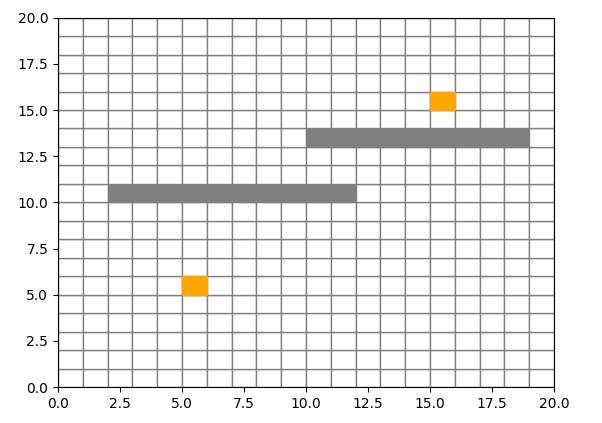本文中，g为已走过的距离，h为期望距离、启发值。文中会频繁使用这两个概念。

A*算法中的h，根据实际地图的拓扑结构，可选用以下三种距离，假设A与B点横纵坐标距离x和y：

1.曼哈顿距离，只允许4个方向移动，AB的距离为：x + y；

2.对角距离，允许8方向移动，AB的距离为：x + y + (sqrt(2)-2)*min(x, y)；

3.欧几里得距离，允许任意方向移动，AB的距离为：sqrt(pow2(x)+pow2(y))；

网格结构常用的便是8方向移动，所以我们这边会选择对角距离作为h。

## 数据结构

我在处理程序问题一般是：先定义数据结构，然后再补充算法本体。

我们这次先从底层的数据结构逐级往上定义。从点、路径到整个地图（我这边只展示关键的数据结构代码）：

# 点的定义
class Vector2:
x = 0
y = 0

def __init__(self, x, y):
self.x = x
self.y = y

# 树结构，用于回溯路径
class Vector2Node:
pos = None  # 当前的x、y位置
frontNode = None    # 当前节点的前置节点
childNodes = None   # 当前节点的后置节点们
g = 0   # 起点到当前节点所经过的距离
h = 0   # 启发值
D = 1

def __init__(self, pos):
self.pos = pos
self.childNodes = []

def f(self):
return self.g + self.h

# 地图
class Map:
map = None  # 地图，0是空位，1是障碍
startPoint = None   # 起始点
endPoint = None # 终点

tree = None # 已经搜寻过的节点，是closed的集合
foundEndNode = None # 寻找到的终点，用于判断算法结束

def __init__(self, startPoint, endPoint):
self.startPoint = startPoint
self.endPoint = endPoint
row = *MAP_SIZE
self.map = []
for i in range(MAP_SIZE):
self.map.append(row.copy())

# 判断当前点是否超出范围
def isOutBound(self, pos):
return pos.x < 0 or pos.y < 0 or pos.x >= MAP_SIZE or pos.y >= MAP_SIZE

# 判断当前点是否是障碍点
def isObstacle(self, pos):
return self.map[pos.y][pos.x] == 1

# 判断当前点是否已经遍历过
def isClosedPos(self, pos):
if self.tree == None:
return False
nodes = []
nodes.append(self.tree)
while len(nodes) != 0:
node = nodes.pop()
if node.pos == pos:
return True
if node.childNodes != None:
for nodeTmp in node.childNodes:
nodes.append(nodeTmp)
return False


PS.我们这边使用matplotlib作为图像输出，具体怎么使用或怎么使其更好看可以参考源代码或第一篇参考文章。

## 算法实现

A*算法的大概思路是：

1.从起始点开始遍历周围的点（专业点的说法是放到了一个open集合中，而我们这边的变量名叫做willProcessNodes）；

2.从open集合中寻找估值，即使用Vector2Node.f()函数计算的值，最小的点作为下一个遍历的对象；

3.重复上面的步骤，直到找到了终点。

具体实现：

# 地图
class Map:
def process(self):
# 初始化open集合，并把起始点放入
willProcessNodes = deque()
self.tree = Vector2Node(self.startPoint)
willProcessNodes.append(self.tree)

# 开始迭代，直到找到终点，或找完了所有能找的点
while self.foundEndNode == None and len(willProcessNodes) != 0:
# 寻找下一个最合适的点，这里是最关键的函数，决定了使用什么算法
node = self.popLowGHNode(willProcessNodes)

# 获取合适点周围所有的邻居
neighbors = self.getNeighbors(node.pos)
for neighbor in neighbors:
# 初始化邻居，并计算g和h
childNode = Vector2Node(neighbor)
childNode.frontNode = node
childNode.calcGH(self.endPoint)
node.childNodes.append(childNode)

# 添加到open集合中
willProcessNodes.append(childNode)

# 找到了终点
if neighbor == self.endPoint :
self.foundEndNode = childNode

# 广度优先，直接弹出先遍历到的节点
def popLeftNode(self, willProcessNodes):
return willProcessNodes.popleft()

# dijkstra，寻找g最小的节点
def popLowGNode(self, willProcessNodes):
foundNode = None
for node in willProcessNodes:
if foundNode == None:
foundNode = node
else:
if node.g < foundNode.g:
foundNode = node
if foundNode != None:
willProcessNodes.remove(foundNode)
return foundNode

# A*，寻找f = g + h最小的节点
def popLowGHNode(self, willProcessNodes):
foundNode = None
for node in willProcessNodes:
if foundNode == None:
foundNode = node
else:
if node.f() < foundNode.f():
foundNode = node
if foundNode != None:
willProcessNodes.remove(foundNode)
return foundNode


我们可以看到在寻找点的时候使用了popLowGHNode，这是使用A*的关键函数，可以替换成上面两个函数使用不同的算法。以下展示使用不同算法的效果。

广度优先遍历（绿点代表遍历过的，蓝点代表路径结果）：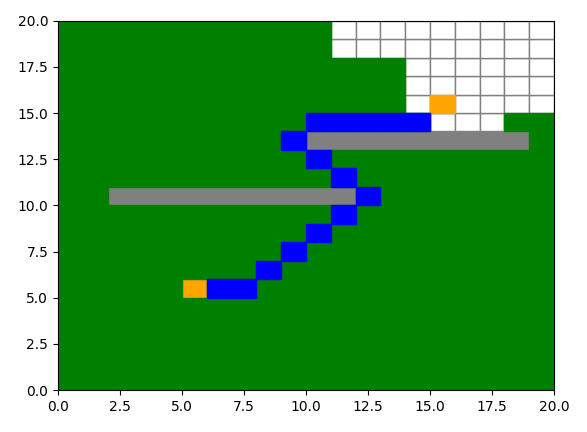Dijkstra算法：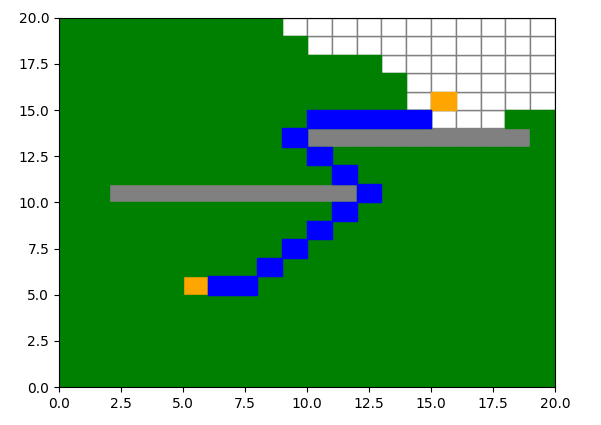A*算法：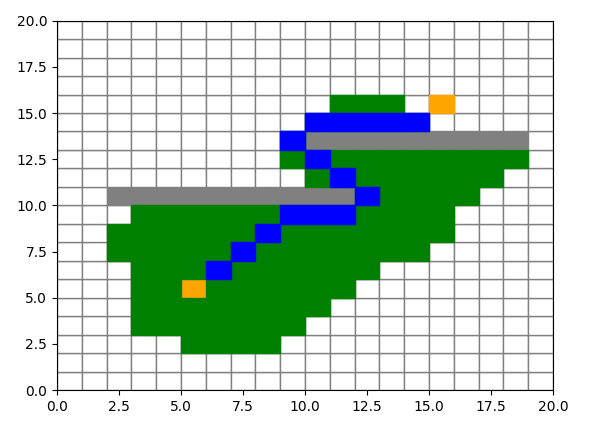在A*的实现中，h的计算也是个重要的参数，像是本文上面中使用真实的预估距离作为h，为了方便我们称该值为d：

1.h = 0，即Dijkstra算法；

2.h < d，预估值有一定的用处，但相比 h = d 而言性能较差；

3.h = d，性能最优，并且能找到最佳路径；

4.h > d，性能可能进一步优化（也可能更差），但不一定是最优路径；

5.h >> g，变成了最佳优先搜索。

可以尝试调节Vector2Node.D查看效果。

## 参考

路径规划之 A* 算法 - 知乎

Python3 教程 | 菜鸟教程

A*算法_百度百科

《游戏人工智能编程案例精粹》

展开全文python 寻路 AI
•    本系列文章主要介绍基于A*算法的路径规划的实现，并使用MATLAB进行仿真演示。    一、 A*算法简介       A*（A-Star)算法是一种静态路网中求解最短路径最有效的直接搜索方法，也是解决许多搜索问题的...

## -----------------------------------------------------------------------------

### 一、前言（本系列文章简介）

#### 我们先来看一下前两篇文章介绍的内容我们要完成的效果（也就是没有经过任何优化的效果，优化后的效果见本系列第三篇文章），我们要在随机生成的环境中（障碍物的位置，起始点，终止点均随机生成），寻找从起始点到达终止点的路径，如果该路径存在，则将其绘制出来，其效果如下：### 1、环境边长（方格数）的设定以及障碍物占总方格数的比例的设定

          n = 20;   % 产生一个n x n的方格，修改此值可以修改生成图片的方格数（也就是在多大的环境内进行路径规划）
wallpercent = 0.4;  % 这个变量代表生成的障碍物占总方格数的比例 ，如0.4 表示障碍物占总格数的40%



### 2、随机方格、障碍物、起始点和终止点 创建函数的编写

#### 这个函数的作用就是生成n x n的矩阵，矩阵中的信息表明该位置是否有障碍物，是否是起始点或者终止点

##### （1）生成一个n x n的单位矩阵，并在此基础上加上一个随机数（rand函数用于生成在0到1范围内的随机数）
         field = ones(n,n) + 10*rand(n,n);%生成一个n*n的单位矩阵+0到10范围内的一个随机数

##### （2）随机选取障碍物的位置，并将其值设为Inf
        field(ind2sub([n n],ceil(n^2.*rand(n*n*wallpercent,1)))) = Inf;%向上取整

##### 运行一下以上介绍的代码，生成的矩阵如下所示，（矩阵中Inf表示此处有障碍物）：##### （3）随机生成起始点和终止点
             startposind = sub2ind([n,n],ceil(n.*rand),ceil(n.*rand));
goalposind = sub2ind([n,n],ceil(n.*rand),ceil(n.*rand));
field(startposind) = 0; field(goalposind) = 0;  %把矩阵中起始点和终止点处的值设为0

##### （4）生成一个新的nxn矩阵，将起始点设为0，其他位置设为NaN（这个矩阵的作用后续用到时再介绍）
            costchart = NaN*ones(n,n);%生成一个nxn的矩阵costchart，每个元素都设为NaN。就是矩阵初始NaN无效数据
costchart(startposind) = 0;%在矩阵costchart中将起始点位置处的值设为0


##### （5）生成一个nxn的元胞数组，将障碍物处设为0，起始点处设为 ‘S’，终止点处设为’G’（这个元胞数组的作用后续用到时再介绍）
            fieldpointers = cell(n,n);%生成元胞数组n*n
fieldpointers{startposind} = 'S';
fieldpointers{goalposind} = 'G'; %将元胞数组的起始点的位置处设为 'S'，终止点处设为'G'
fieldpointers(field==inf)={0};

##### 到目前为止2个矩阵和元胞数组内数据如下（其中一种随机情况）：##### （6）我们将以上的代码封装成一个函数取名为initializeField，该函数的输入量为n和wallpercent，输出量为field, startposind, goalposind, costchart, fieldpoin ters，如下所示：
     function [field, startposind, goalposind, costchart, fieldpointers] = ...
initializeField(n,wallpercent)
field = ones(n,n) + 10*rand(n,n);%生成一个n*n的单位矩阵+0到10范围内的一个随机数
field(ind2sub([n n],ceil(n^2.*rand(n*n*wallpercent,1)))) = Inf;%向上取整

% 随机生成起始点和终止点
startposind = sub2ind([n,n],ceil(n.*rand),ceil(n.*rand));  %随机生成起始点的索引值
goalposind = sub2ind([n,n],ceil(n.*rand),ceil(n.*rand));   %随机生成终止点的索引值
field(startposind) = 0; field(goalposind) = 0;  %把矩阵中起始点和终止点处的值设为0

costchart = NaN*ones(n,n);%生成一个nxn的矩阵costchart，每个元素都设为NaN。就是矩阵初始NaN无效数据
costchart(startposind) = 0;%在矩阵costchart中将起始点位置处的值设为0

% 生成元胞数组
fieldpointers = cell(n,n);%生成元胞数组n*n
fieldpointers{startposind} = 'S'; fieldpointers{goalposind} = 'G'; %将元胞数组的起始点的位置处设为 'S'，终止点处设为'G'
fieldpointers(field==inf)={0};

end


### 3、将随机生成的方格及障碍物的数据生成图像

##### （1）figure图像的初始化
      if isempty(gcbf)
axes('position', [0.01 0.01 0.99 0.99]);
else
gcf; cla;
end

##### 这段代码的效果如下：##### （2）将field矩阵中的随机数设为0
    n = length(field);  %获取矩阵的长度，并赋值给变量n
field(field < Inf) = 0; %将fieid矩阵中的随机数（也就是没有障碍物的位置处）设为0##### 先回顾一下，之前我们通过initializeField函数，生成的field矩阵中，障碍物的位置处设为Inf，没有障碍物的位置处为1到11的随机数，如上图所示，现在我们将没有障碍物的地方的随机数也设为0，结果如下图所示（因为每次程序运行生成的矩阵信息都是随机的，所以与上图并不是一一对应的关系）：##### （3）利用pcolor（）函数生成彩色方格
 pcolor(1:n+1,1:n+1,[field field(:,end); field(end,:) field(end,end)]);%多加了一个重复的（由n X n变为 n+1 X n+1 ）

##### 那么怎么扩展呢，这就需要先了解一下矩阵的串联，直接用举例子的方式来介绍吧，如果串联的矩阵之间是空格或者逗号，则横向串联，如果串联的矩阵之间是分号则纵向串联，如下所示##### 了解了矩阵的串联，那么我们返回来理解[field field(:,end); field(end,:) field (end,end)]就容易了很多，这个无非就是在原有的矩阵field基础上，将其最后一行和最后一列再串到矩阵中去（也就是相当于复制了），结果如下：##### 运行一下程序看一下效果：##### 接下来我们来介绍一下matlab里的colormap函数 ，matlab画图时，如果想将不同的值用不同的颜色表示，可以使用colormap这个函数，我们知道索引图像有两个分量，一个是数据矩阵X，一个是彩色映射矩阵map，colormap就是用来设定map的函数。MATLAB中默认自带了18种colormap，最常用的jet图像如下所示：##### colormap实际上是一个mx3的矩阵，每一行的3个值都为0-1之间数，分别代表颜色组成的rgb值，如[0 0 1]代表蓝色。在了解了以上内容后我们再来看以下语句（flipud函数用于实现矩阵的上下翻转）：
            cmap = flipud(colormap('jet'));

##### 生成的cmap是一个256X3的矩阵，每一行的3个值都为0-1之间数，分别代表颜色组成的rgb值cmap(1,:) = zeros(3,1); cmap(end,:) = ones(3,1); %将矩阵cmap的第一行设为0 ，最后一行设为1
colormap(flipud(cmap)); %进行颜色的倒转

##### （4）在方格中添加起始点和终止点

    hold on;
axishandle = pcolor([1:n+1],[1:n+1],[costchart costchart(:,end); costchart(end,:)       costchart(end,end)]);  %将矩阵costchart进行拓展，插值着色后赋给axishandle
[goalposy,goalposx] = ind2sub([n,n],goalposind);
[startposy,startposx] = ind2sub([n,n],startposind);
plot(goalposx+0.5,goalposy+0.5,'ys','MarkerSize',10,'LineWidth',6);
plot(startposx+0.5,startposy+0.5,'go','MarkerSize',10,'LineWidth',6);
uicontrol('Style','pushbutton','String','RE-DO', 'FontSize',12, ...
'Position', [1 1 60 40], 'Callback','astardemo');

##### （5）将本部分内容封装成一个函数createFigure，输入参数为field,costchart, startposind,goalposind，输出参数为axishandle 。

function axishandle = createFigure(field,costchart,startposind,goalposind)

% 这个if..else结构的作用是判断如果没有打开的figure图，则按照相关设置创建一个figure图
if isempty(gcbf)                                       %gcbf是当前返回图像的句柄，isempty(gcbf)假如gcbf为空的话，返回的值是1，假如gcbf为非空的话，返回的值是0
figure('Position',[450 50 700 700], 'MenuBar','none');  %对创建的figure图像进行设置，设置其距离屏幕左侧的距离为450，距离屏幕下方的距离为50，长度和宽度都为700，并且关闭图像的菜单栏
axes('position', [0.01 0.01 0.99 0.99]);               %设置坐标轴的位置，左下角的坐标设为0.01,0.01   右上角的坐标设为0.99 0.99  （可以认为figure图的左下角坐标为0 0   ，右上角坐标为1 1 ）
else
gcf; cla;   %gcf 返回当前 Figure 对象的句柄值，然后利用cla语句来清除它
end

n = length(field);  %获取矩阵的长度，并赋值给变量n
field(field < Inf) = 0; %将fieid矩阵中的随机数（也就是没有障碍物的位置处）设为0
pcolor(1:n+1,1:n+1,[field field(:,end); field(end,:) field(end,end)]);%多加了一个重复的（由n X n变为 n+1 X n+1 ）

cmap = flipud(colormap('jet'));  %生成的cmap是一个256X3的矩阵，每一行的3个值都为0-1之间数，分别代表颜色组成的rgb值
cmap(1,:) = zeros(3,1); cmap(end,:) = ones(3,1); %将矩阵cmap的第一行设为0 ，最后一行设为1
colormap(flipud(cmap)); %进行颜色的倒转
hold on;

axishandle = pcolor([1:n+1],[1:n+1],[costchart costchart(:,end); costchart(end,:) costchart(end,end)]);  %将矩阵costchart进行拓展，插值着色后赋给axishandle

[goalposy,goalposx] = ind2sub([n,n],goalposind);
[startposy,startposx] = ind2sub([n,n],startposind);
plot(goalposx+0.5,goalposy+0.5,'ys','MarkerSize',10,'LineWidth',6);
plot(startposx+0.5,startposy+0.5,'go','MarkerSize',10,'LineWidth',6);
uicontrol('Style','pushbutton','String','RE-DO', 'FontSize',12, ...
'Position', [1 1 60 40], 'Callback','astardemo');
end



#### 到这里第二大部分环境的创建就完成了，我们通过编写的initializeField函数和createFigure函数完成了环境的创建，到目前为止的完整的代码如下：

clc;             %清除命令窗口的内容
clear all;       %清除工作空间的所有变量，函数，和MEX文件
close all;       %关闭所有的figure窗口

n = 20;   % 产生一个n x n的方格，修改此值可以修改生成图片的方格数
wallpercent = 0.4;  % 这个变量代表生成的障碍物占总方格数的比例 ，如0.5 表示障碍物占总格数的50%

[field, startposind, goalposind, costchart, fieldpointers] =initializeField(n,wallpercent);
createFigure(field,costchart,startposind,goalposind)

%%
function [field, startposind, goalposind, costchart, fieldpointers] = ...
initializeField(n,wallpercent)
field = ones(n,n) + 10*rand(n,n);%生成一个n*n的单位矩阵+0到10范围内的一个随机数
field(ind2sub([n n],ceil(n^2.*rand(n*n*wallpercent,1)))) = Inf;%向上取整
% 随机生成起始点和终止点
startposind = sub2ind([n,n],ceil(n.*rand),ceil(n.*rand));  %随机生成起始点的索引值
goalposind = sub2ind([n,n],ceil(n.*rand),ceil(n.*rand));   %随机生成终止点的索引值
field(startposind) = 0; field(goalposind) = 0;  %把矩阵中起始点和终止点处的值设为0

costchart = NaN*ones(n,n);%生成一个nxn的矩阵costchart，每个元素都设为NaN。就是矩阵初始NaN无效数据
costchart(startposind) = 0;%在矩阵costchart中将起始点位置处的值设为0

% 生成元胞数组
fieldpointers = cell(n,n);%生成元胞数组n*n
fieldpointers{startposind} = 'S'; fieldpointers{goalposind} = 'G'; %将元胞数组的起始点的位置处设为 'S'，终止点处设为'G'
fieldpointers(field==inf)={0};

end

%%

function axishandle = createFigure(field,costchart,startposind,goalposind)

% 这个if..else结构的作用是判断如果没有打开的figure图，则按照相关设置创建一个figure图
if isempty(gcbf)                                       %gcbf是当前返回图像的句柄，isempty(gcbf)假如gcbf为空的话，返回的值是1，假如gcbf为非空的话，返回的值是0
figure('Position',[450 100 700 700], 'MenuBar','none');  %对创建的figure图像进行设置，设置其距离屏幕左侧的距离为450，距离屏幕下方的距离为50，长度和宽度都为700，并且关闭图像的菜单栏
axes('position', [0.01 0.01 0.99 0.99]);               %设置坐标轴的位置，左下角的坐标设为0.01,0.01   右上角的坐标设为0.99 0.99  （可以认为figure图的左下角坐标为0 0   ，右上角坐标为1 1 ）
else
gcf; cla;   %gcf 返回当前 Figure 对象的句柄值，然后利用cla语句来清除它
end

n = length(field);  %获取矩阵的长度，并赋值给变量n
field(field < Inf) = 0; %将fieid矩阵中的随机数（也就是没有障碍物的位置处）设为0
pcolor(1:n+1,1:n+1,[field field(:,end); field(end,:) field(end,end)]);%多加了一个重复的（由n X n变为 n+1 X n+1 ）

cmap = flipud(colormap('jet'));  %生成的cmap是一个256X3的矩阵，每一行的3个值都为0-1之间数，分别代表颜色组成的rgb值
cmap(1,:) = zeros(3,1); cmap(end,:) = ones(3,1); %将矩阵cmap的第一行设为0 ，最后一行设为1
colormap(flipud(cmap)); %进行颜色的倒转
hold on;

axishandle = pcolor([1:n+1],[1:n+1],[costchart costchart(:,end); costchart(end,:) costchart(end,end)]);  %将矩阵costchart进行拓展，插值着色后赋给axishandle

[goalposy,goalposx] = ind2sub([n,n],goalposind);
[startposy,startposx] = ind2sub([n,n],startposind);
plot(goalposx+0.5,goalposy+0.5,'ys','MarkerSize',10,'LineWidth',6);
plot(startposx+0.5,startposy+0.5,'go','MarkerSize',10,'LineWidth',6);

uicontrol('Style','pushbutton','String','RE-DO', 'FontSize',12, ...
'Position', [1 1 60 40], 'Callback','astardemo');
end



#### 运行结果如下（黑色的方格表示该处有障碍物，绿色圆环表示起点，黄色方块表示终点）：：本篇文章到这里就结束了，欢迎大家继续阅读本系列文章的后续文章，本文介绍的内容的完整代码的MATLAB文件我会放到附件里，听说在上传的时候设为粉丝可下载是不需要花费积分的，大家看一下需不需要积分，若还是需要积分，在评论区留言，我直接传给你

本篇文章内容的附件链接：A星算法路径规划博文附件

欢迎大家积极交流，本文未经允许谢绝转载

展开全文matlab 路径规划 代码逐行解释
• 之前在做rpg游戏的时候实体移动用的是A*算法，那时候没有去研究A*算法原理，前天看了一篇博客介绍A*算法，按照自己的理解记录一下A*算法。 参考：https://blog.csdn.net/nie2314550441/article/details/106673966 ...深度优先
• ## A*算法详解

万次阅读 多人点赞 2020-06-10 20:06:33
A* 寻路算法 原文地址：http://www.gamedev.net/reference/articles/article2003.asp 概述 虽然掌握了A*算法的人认为它容易，但是对于初学者来说，A*算法还是很复杂的。 搜索区域(The Search Area) 我们假设...
• A*算法A*（A-Star)算法是一种静态路网中求解最短路径最有效的直接搜索方法，也是解决许多搜索问题的有效算法算法中的距离估算值与实际值越接近，最终搜索速度越快。 定义解析 A*算法是一个“搜索算法”，实质...python 人工智能
• A*算法解决传教士与野人过河问题 * 程 序 说 明 * * 功能: 用A*算法求解传教士与野人问题。M=C=5, K=3 * * 说明: * * 本程序按照《人工智能导论》一书所介绍的A*算法求解传教士与野人问题。 * * * * 注意:...
• 基于状态空间表示法的搜索算法解决八数码难题 本文的pdf文件链接：link 一、问题重述 1.1 背景介绍        如今处于人工智能和大数据时代，每天都有成千上万的数据产生，而我们...人工智能 python
• A*算法原理及例题介绍一、A*算法的基本原理二、A*算法例题介绍三、例题理论分析四、程序流程图五、启发式函数h(n)的定义六、A*搜索过程中的节点信息七、A*算法与A算法、宽度优先的区别 一、A*算法的基本原理 （A-...
• 设起始节点为 s(start），终点为g(goal)，首先从A算法出发。 一、评价函数 A算法的评价函数：f(n) = g(n) + h(n)​​​​​​ g*(n)：从s到n的最短路径的耗散值 h*(n)：从n到g的最短路径的耗散值 注意这里的带...
• A算法： 利用评价函数来选择下一个节点。 图引用自 -北京联合大学 彭涛老师在 中国慕课的 《人工智能概论》。 估价函数没有定论，可以有不同方法。 这里采用处在错误位置的数字的数量。 代码在： github 一...八数码问题
• ## A*算法原理概述

万次阅读 多人点赞 2019-05-06 19:57:27
这里是目录路径规划A*算法原理路线规划理论概述Dijkstra算法最佳优先搜索算法A*算法理论概述 路径规划A*算法原理 路线规划理论概述 移动一个物体直观上很容易的，但是物品的路线规划是复杂的。如下图3-1所示，物体...
• A*算法求解迷宫寻路问题 设置两种地图，两种启发式函数，分析不同起点终点，分析不同启发式函数，分析不同地图。机器学习 ai
• ## A*算法

万次阅读 2018-08-21 10:07:28
A*算法常用于二维地图路径规划,算法所采用的启发式搜索可以利用实际问题所具备的启发式信息来指导搜索,从而减少搜索范围,控制搜索规模,降低实际问题的复杂度 。 二、算法原理 A*算法的原理是设计一个代价估计函数:...
• 这里写自定义目录标题移动机器人路径规划算法介绍及A*算法详解路径规划算法总结各种算法比较A*算法详解 移动机器人路径规划算法介绍及A*算法详解 随着人工智能的兴起，移动机器人逐渐走入了更多人的视线，ML和DL为...
• ## A*算法简介-matlab篇

千次阅读 多人点赞 2020-02-07 10:57:33
如果你对A*了如指掌，并且是算法大师，这篇文章帮不到你，请指教一下。 我接下来基本上所有文章都是针对Atsushi Sakai这个作者在GitHub上发布的，添加注释以及自己的一些思考，希望可以帮助到一些新入门的人。希望...matlab
• 上一篇文章在原有的A*算法上增加了权值，解决了混合型地图的最短时间循迹问题。本篇文章我们来讨论一下A*算法，在三维地图上可行性。 可行性讨论 在二维空间上，我们把地图分割成许许多多大小一致的正方格。而在三维...
• 一、Dijkstra算法A*算法比较 Dijkstra算法A*算法总结 Dijkstra算法计算源点到其他所有点的最短路径长度，A*关注点到点的最短路径(包括具体路径)。 Dijkstra算法建立在较为抽象的图论层面，A*算法可以更轻松地...python
• ## A*算法（二）启发式算法

万次阅读 多人点赞 2019-09-03 23:20:50
启发式函数的使用、权衡代价函数、衡量单位、精确的启发式函数、预计算的精确启发式函数、线性精确启发式算法、网格地图中的启发式算法、曼哈顿距离、对角线距离、欧几里得距离、平方后的欧几里得距离、Breaking ...曼哈顿距离
• ## 双向A*算法

千次阅读 2020-11-16 07:44:23
可参考：双向A*算法浅析 代码理解可主要看下这个： 算法：Astar寻路算法改进，双向A*寻路算法
• ## c语言实现a*算法

千次阅读 2019-12-22 11:23:05
最近要实训需要实现迷宫，所以就先实现了之前一直总是了解算法思路的a算法。最开始我是想凭借自己对于这个寻路算法的理解，完全的按照自己的思路实现，最后结果感人，在实现函数的传递时候，才发现自己对于c语言函数...
• ## A*算法详解（讲的一级棒 ）

万次阅读 多人点赞 2018-08-23 16:03:28
虽然掌握了 A* 算法的人认为它容易，但是对于初学者来说， A* 算法还是很复杂的。 搜索区域(The Search Area) 我们假设某人要从 A 点移动到 B 点，但是这两点之间被一堵墙隔开。如图 1 ，绿色是 A ，红色是 B ，...
• ## A*算法的Matlab实现

万次阅读 多人点赞 2019-03-26 22:40:33
A*算法的Matlab实现A*算法的Matlab实现一、参考博客二、代码如下：1.pathfinding.m2.AStar.m3.FindList.m4.GetBoundary.m5.GetObstacle.m6.GetPath7.h.m8.isObstacle9.isopen10.MotionModel11.plot_map.m ...
• ## A*算法的C#实现

千次阅读 2018-09-01 21:04:55
本文的主要内容是讲述A *寻路算法的基本原理，实现步骤以及对应的C＃代码，适合读者用于学习A *算法或 使用此代码提供的接口完成游戏中的寻路功能。  详细的A *算法的原理，请参照：https://blog.csdn.net/d...网格寻路...